## General Question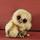# How do I solve this rational equation word problem?

Asked by chelle21689 (6995) October 25th, 2010

I’m having a very hard time figuring this out.
“Two joggers, one averaging 8mph and one averaging 6mph, start from a designated initial point. The slower jogger arrives at the end of the run a half hour after the other jogger. Find the distance of the run.

This is how I set it up.
8X=6X+180
Then, I cross multiply. I get the wrong answer though. The answer is 12 miles.

Observing members: 0Composing members: 0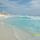I am no expert, but I believe you missed something. You do not tell how long anyone ran? The second jogger arrives an half hour after what? After 24 hours, 10 hours?
I am confused, sorry I can not help at this point.

MissPoovey (893)“Great Answer” (0) Flag as…I didn’t miss anything. This is the word problem the book gives. lol

chelle21689 (6995)“Great Answer” (0) Flag as…Hmmm, I hope someone smarter than me answers then, sorry.
Where did you get the 180?

MissPoovey (893)“Great Answer” (0) Flag as…Response moderated (Off-Topic)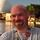Pace = Distance / Time

P1 = 8mph
P2 = 6mph

D = D1 = D2

T2 = T1 + 0.5

P2 = D2 / T2
6 = D / (T1 + 0.5)
P1 = D1 / T1
8 = D / T1

Now we have two equations, two variables (D and T1)

Solve Equation 2 for D
D = 8 * T1
Plug D into Equation 1

6 = (8 * T1) / (T1 + 0.5)
6T1 + 3 = 8T1
3 = 2T1
T1 = 1.5

Plug T1=1.5 back into second equation
D = 8 * 1.5
D = 12

Therefore, the distance is 12 miles. The Faster runner completed it in 1.5 hours, and the slower runner completed it in (T2 = T1 + 0.5 = 2 hours

MrItty (17371)“Great Answer” (2) Flag as…Response moderated (Off-Topic)AHH! I got it!!! LoL!

chelle21689 (6995)“Great Answer” (0) Flag as…Response moderated (Off-Topic)20/x=16/x-2

I got the first rate… cross multiply and it’s 10mph!

Now for the second one. I must work at it.

chelle21689 (6995)“Great Answer” (0) Flag as…Response moderated (Writing Standards)I solved a diff problem I’ve been having trouble with lol

chelle21689 (6995)“Great Answer” (1) Flag as…Thanks Mritty!!!!!!

chelle21689 (6995)“Great Answer” (0) Flag as…Where did you get .5 from?

chelle21689 (6995)“Great Answer” (0) Flag as…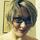@chelle21689 Since the speed in in hours, you can’t use minutes on the other side of the equation. Half hour translates to .5

Simone_De_Beauvoir (38956)“Great Answer” (0) Flag as…@chelle21689 0.5 = one half. The pace is in miles per hour. Therefore your time has to be in hours as well. One jogger was one half hour faster than the other.

MrItty (17371)“Great Answer” (0) Flag as…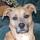Let “T” = the time run for the faster runner in hours.

Then his distance run is 8 mph x T, or 8T miles.
The time for the slower runner is T + .5; his distance is represented by 6mph x (T + .5) or 6 * (T + .5) or 6T + 3

You now have two terms that equate to each other, since the distances each runner ran are equal.
8T = 6T + 3
2T = 3
T = 1.5 hours

Distance for the first runner = 8mph x 1.5 hours = 12 miles
To check that, solve for the distance that the second runner ran, using those terms.

CyanoticWasp (20063)“Great Answer” (2) Flag as…Let L= the lead that the faster runner has at the end of the race
Let t = time that the faster runner runs
Let d = total distance

L=distance slower runner runs in ½ hr = 6mph*½hr= 3 miles

L is also the lead that the faster runner builds up over the course of the race.
L=3mi = (8–6)mph* t, so t=1.5 hr

d=8mph *1.5 hr=12 mi

LostInParadise (25507)“Great Answer” (1) Flag as…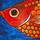Or….
If X is the distance run
The faster runner will take X/8 hours to run this far
The slower runner will take X/6 hours
The difference is ½ hours so X/6 – X/8 = ½
Therefore X = 12

flutherother (29282)“Great Answer” (2) Flag as…Thanks guys I really appreciate it.

chelle21689 (6995)“Great Answer” (0) Flag as…or